Vectors and conics

This free course is available to start right now. Review the full course description and key learning outcomes and create an account and enrol if you want a free statement of participation.

Free course

1.7 Planes in three-dimensional Euclidean space

We now look at the general form of the equation of a plane in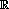3.

Three planes whose equations are easy to find are those that contain a pair of axes. For example, the (x, y)-plane is the plane that contains the x-axis and the y-axis. Points which lie in this plane are precisely those points (x, y, z) in3 for which z = 0, so the equation of the (x, y)-plane is z = 0.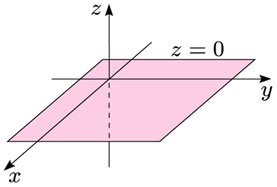Example 7

Find the equations of the (y, z)-plane and the (x, z)-plane.

Points (x, y, z) that lie in the (y, z)-plane all have x = 0; so x = 0 is the equation of this plane.

Similarly, points (x, y, z) that lie in the (x, z)-plane all have y = 0; so y = 0 is the equation of this plane.

Example 8

Sketch the planes whose equations are as follows.

• (a) z = 2

• (b) y = −1

• (a)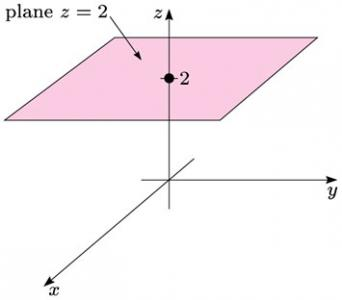• (b)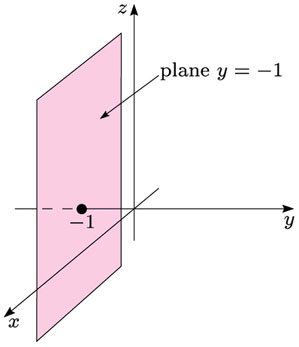You saw in Section 1.2 that the general form of the equation of a line in2 is ax + by = c, where a, b and c are real, and a and b are not both zero. In3 the analogue of this equation is the equation of a plane.

We have already met the planes with equations x = 0, y = 0 and z = 0. Each of these equations is a special case of a more general equation ax + by + cz = d , where a, b, c and d are real, and a, b and c are not all zero. In fact, the equation of any plane in3 is of this form.

Equation of a plane

The general equation of a plane in3 is ax + by + cz = d, where a, b, c and d are real, and a, b and c are not all zero.

(We shall prove this in Section 3.3).

Example 3

Determine the general form of the equation of a plane that passes through the origin.

Let the plane have equation

ax + by + cz = d

Since (0, 0, 0) lies in the plane, its coordinates must satisfy the equation of the plane; thus

a × 0 + b × 0 + c × 0 = d,

so d = 0. Also, the plane whose equation is

ax + by + cz = 0

clearly passes through the origin (0, 0, 0).

Hence the general form of the equation of a plane that passes through the origin is

ax + by + cz = 0

where a, b and c are real and not all zero.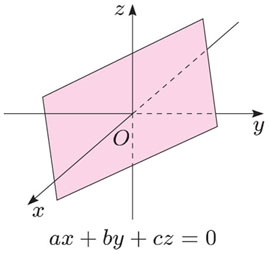The (x, y)-, (y, z)- and (x, z)-planes all pass through the origin, and their equations are all of the form shown above.

Example 9

For each of the following points, determine the general form of the equation of a plane that passes through the point.

• (a) (1, 2, 3)

• (b) (−1, −4, 2)

• (a) Let the equation of the plane be of the form

• ax + by + cz = d

• Since (1, 2, 3) lies in the plane, its coordinates must satisfy the equation of the plane; thus

• a × 1 + b × 2 + c × 3 = d,

• so

• d = a + 2b + 3c.

• Thus the general form of the equation of a plane through (1, 2, 3) is

• ax + by + cz = a + 2b +3c

• (b) Let the equation of the plane be of the form

• ax + by + cz = d

• Since (−1, −4, 2) lies in the plane, its coordinates must satisfy the equation of the plane; thus

• a × (−1) + b × (−4) + c × 2 = d,

• so

• d = −a - 4b + 4c.

• Thus the general form of the equation of a plane through (−1, −4, 2) is

• ax + by + cz = −a − 4b + 2c.

M208_1